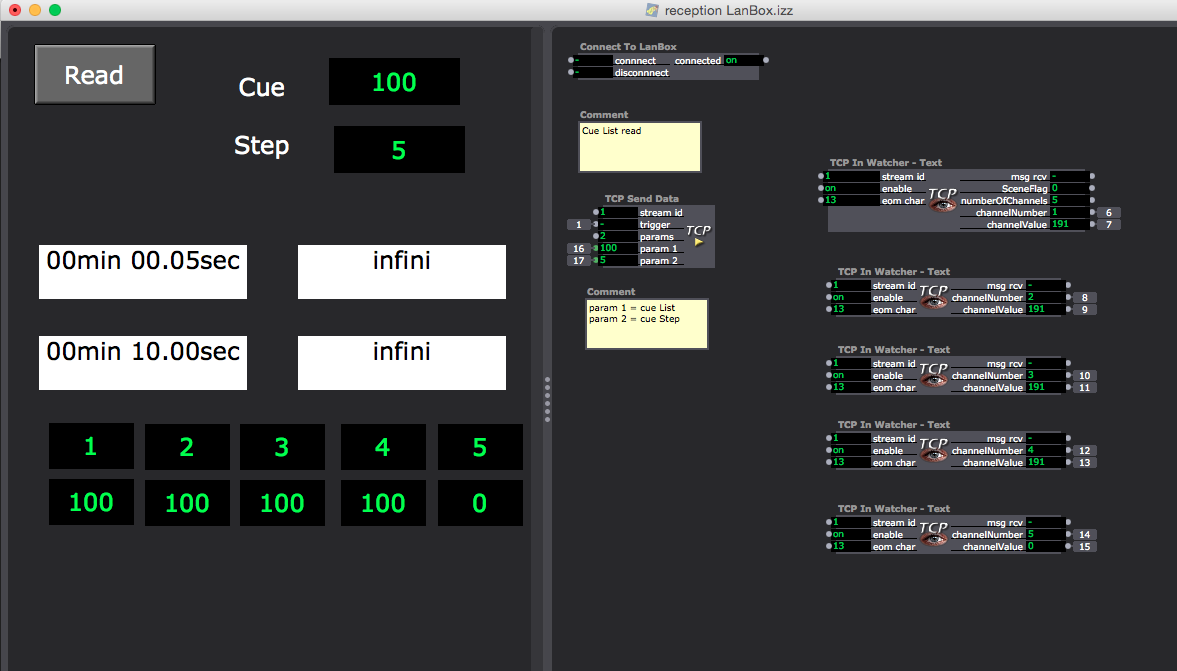# TCP In Watcher - Text question

• Hello, I am working on a patch allowing dialogue with lanbox. By example, if I need the list of channel value in a Cue step, I send with TCP Send Data "* AD 00 A8 02 #" to lanbox and I have an answer. The problem is the length of the answer is variable, depending on how channel are defined in the cue step.
For reception, If I use that in TCP In Watcher - Text actor:
"" SceneFlag:int=2x numberOfChannels:int=4x channelNumber:int=4x channelValue:int=2x channelNumber:int=4x channelValue:int=2x channelNumber:int=4x channelValue:int=2x channelNumber:int=4x channelValue:int=2x channelNumber:int=4x channelValue:int=2x
If I have 5 or less channel active, it works, if I have more, there is no result.
My first solution is to use independent receptor for each channel:
first: "
" SceneFlag:int=2x numberOfChannels:int=4x channelNumber:int=4x channelValue:int=2x
second: "?????????????" channelNumber:int=4x channelValue:int=2x
third: "???????????????????" channelNumber:int=4x channelValue:int=2x
fourth: "?????????????????????????" channelNumber:int=4x channelValue:int=2x
etc. But it's not very elegant for 256 channels…
I search a solution to
– have conditional output for TCP in watcher
– or to have a raw output of TCP in watcher as to compute it in javascript and output the value of each channel.
If someone could help?
Thank you,
Jacques• you can use:

msg:string={00-FF}
to return all values.
That might allow you to get a string you can parse with Javascript.

• Thank you DusX, that helps me a lot, now I have to parse and calculate those bloody hexa characters…

• With the "*AD" command you also get the number of channels. I include an actor, LanboxSceneFader, which uses a cuestep as playback information. Here I read the number of channels, then loop through the channels to perform an action. In this case a multiplication with a fader value.

Maybe this gives you an idea for your programming?

• @DusX, for info here is the script I wrote to parse the lanbox cueStep channel info (24 channels here), far more elegant!

thanks a lot, Jacques

function main()

{

var tcpRecept = arguments;

var out = [-1,-1,-1,-1,-1,-1,-1,-1,-1,-1,-1,-1,-1,-1,-1,-1,-1,-1,-1,-1,-1,-1,-1,-1];

var test = tcpRecept.charAt(0);

if (test == "*")

{

var channelNumber = parseInt((tcpRecept.slice(3,7)), 16);

for (i = 0; i < channelNumber; i++)

{

var channel = parseInt((tcpRecept.slice((7+6i),(11+6i))), 16);

var value = parseInt((tcpRecept.slice((11+6i),(13+6i))),16);

out [channel-1] = value;

}

return out;

}

}

• @gunther

Thank you for your advice, I know and I made and i use some actors like that.
The project is more ambitious, as to show all the level in one cueStep, change it individually and record it changed, in blind or show mode. I am on the good way but I need to use javascript because TCP in Watcher doesn't like when there is some empty output (so it it doesn't output anything) and also because TCP Send data accept only 9 parameters (I want to send 64 channel level at the same time).
Jacques

• @jhoepffner

Thanks for sharing...
its exactly these types of solutions that really add to the value of the forum.

• ```function main()
{
var tcpRecept = arguments;
var tranche = arguments;
var out = [];
for (ii = 0; ii < 64; ii++)
{
out[ii] = -1;
}
var test = tcpRecept.charAt(0);
if (test == "*")
{
var channelNumber = parseInt((tcpRecept.slice(3,7)), 16);
for (i = 0; i < channelNumber; i++)
{
var channel = parseInt((tcpRecept.slice((7+6*i),(11+6*i))), 16);
var value = parseInt((tcpRecept.slice((11+6*i),(13+6*i))),16);
out [channel-1] = value;
}
return out;
}
}
```

• that's the new version for 64 channels, a bit more compact